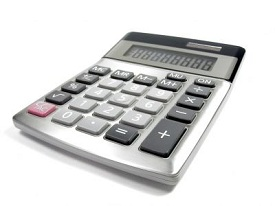Language

## Calculations

### Feed Report Calculations

Commonly used equations for generating feed and forage related calculations are provided below. The information provided in the spreadsheets has not been verified and the accuracy of any of the calculations is not guaranteed. The user takes all responsibility for the use and interpretation of this information.

### Equations for estimating energy from various systems including New York and Pennsylvania

Estimates of Energy Availability, NFTA (estimates of energy availability.mht)

### OARDC Summative Energy Equation of Weiss

OARDC TDN and NEL Spreadsheet
"OARDC Summative Energy Equation"

### NRC 2001 Energy Calucations

NRC 2001 Energy Calculation Spreadsheet
"Energy in the 2001 Dairy NRC: Understanding the System", Jim Linn, University of MN

### Van Amburgh NDF Digestibility Rate Calculations

Van Amburgh Rate Spreadsheet (NDF rate calculation spreadsheet) - Coming Soon
"Corn Silage Neutral Detergent Fiber – Refining a Mathematical Approach for Invitro Rates of Digestion", M. E. Van Amburgh, P. J. Van Soest

### DCAD Calculations